#Using Forms + Controls

Forms in VB.Net are used as containers for Controls. Both Forms + Controls have Properties, Events, + Methods.

In this chapter I'll show you how to use simple Controls to accept + validate user input + display the results of calculations in smart, eye catching + effective user interfaces, + how to create functional user customizable layouts for your forms.

Later in this chapter we'll look at extending Controls through Inheritance, + creating custom Usercontrols to use in your applications.

## This is the first example Project: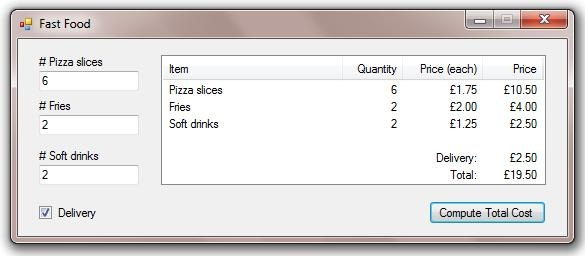I've changed the Name Property for the Form, 3 Textboxes, Checkbox, Listview, + Button.
I've also changed these additional Properties for the Form:

• FormBorderStyle = System.Windows.Forms.FormBorderStyle.FixedSingle
• MaximizeBox = False
• Text = "Fast Food"

And this Property for the Listview:

• View = System.Windows.Forms.View.Detail

Then added 4 columns to the Listview + changed their ColumnHeader text.

Here's the code for the example Project:

```Public Class frmFastFood

Private Sub frmFastFood_Load(ByVal sender As System.Object, ByVal e As System.EventArgs) Handles MyBase.Load
'prompt user to enter some numbers
End Sub

MessageBox.Show("Enter numbers in textboxes", Me.Text, MessageBoxButtons.OK, MessageBoxIcon.Information)
End Sub

Private Sub btnCompute_Click(ByVal sender As System.Object, ByVal e As System.EventArgs) Handles btnCompute.Click
Dim pizza As Integer = 0
Dim fries As Integer = 0
Dim drinks As Integer = 0
Dim delivery As Decimal = If(chkDelivery.Checked, 2.5, 0)

'if all 3 textboxes contain whole numbers...
'display itemized bill in the listview
If Integer.TryParse(txtPizzaSlices.Text, pizza) AndAlso Integer.TryParse(txtFrenchFries.Text, fries) AndAlso Integer.TryParse(txtDrinks.Text, drinks) Then
'this calls the itemize procedure + also calls the computeTotal function as 1 of the parameters
itemize(pizza, fries, drinks, delivery, computeTotal(pizza, fries, drinks, delivery).ToString("c2"))
End If
End Sub

Private Function computeTotal(ByVal pizzaSlices As Integer, ByVal frenchFries As Integer, ByVal softDrinks As Integer, ByVal delivery As Decimal) As Decimal
Return (pizzaSlices * 1.75) + (frenchFries * 2) + (softDrinks * 1.25) + delivery
End Function

Private Sub itemize(ByVal pizzaSlices As Integer, ByVal frenchFries As Integer, ByVal softDrinks As Integer, ByVal delivery As Decimal, ByVal total As String)
'this clears the listview, creates a List(Of ListViewItem) + repopulates the listview
lvBill.Items.Clear()
Dim lvi As New List(Of ListViewItem)

If pizzaSlices > 0 Then lvi.Add(New ListViewItem(New String() {"Pizza slices", pizzaSlices, CDec(1.75).ToString("c2"), CDec(1.75 * pizzaSlices).ToString("c2")}))
If frenchFries > 0 Then lvi.Add(New ListViewItem(New String() {"Fries", frenchFries, CDec(2).ToString("c2"), CDec(2 * frenchFries).ToString("c2")}))
If softDrinks > 0 Then lvi.Add(New ListViewItem(New String() {"Soft drinks", softDrinks, CDec(1.25).ToString("c2"), CDec(1.25 * softDrinks).ToString("c2")}))

If delivery > 0 Then lvi.Add(New ListViewItem(New String() {"", "", "Delivery:", delivery.ToString("c2")}))
lvi.Add(New ListViewItem(New String() {"", "", "Total:", total}))
End Sub

End Class```

## This is the second example Project: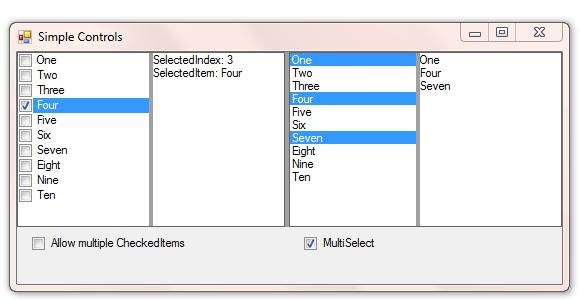This project demonstrates some of the similarities + differences between the CheckedListbox + Listbox controls, + also shows the effects of docking Controls. Run the example project + maximize the Form to see how Docking works. This project also uses SplitContainers which make the Form layout customizable by the user.

I've changed the Form, CheckedListbox, Listbox, 2 Checkboxes, + 2 Textboxes Name Property.

The Form has these additional properties:

• FormBorderStyle = System.Windows.Forms.FormBorderStyle.FixedSingle
• Text = "Simple Controls"

In addition to the obviously visible Controls, there are 3 SplitContainers + a TableLayoutPanel.

The first SplitContainer is Docked to the top of the Form, with each Panel containing another SplitContainer, whose Panels contain a CheckedListbox + a Textbox, + a Listbox + a Textbox.

These are the relevant Properties from the first SplitContainer:

• BackColor = System.Drawing.SystemColors.ControlDark
• BorderStyle = System.Windows.Forms.BorderStyle.FixedSingle
• Dock = System.Windows.Forms.DockStyle.Top
• Location = New System.Drawing.Point(0, 0)

These are the relevant Properties which both child SplitContainers share:

• BackColor = System.Drawing.SystemColors.ControlDark
• Dock = System.Windows.Forms.DockStyle.Fill

The TableLayoutPanel has 1 row + 4 columns. The columns are 2 fixed 12 pixel wide columns, + 2 of 50% of the remaining width.

These are the relevant Properties from the TableLayoutPanel:

• ColumnCount = 4
• Dock = System.Windows.Forms.DockStyle.Top
• RowCount = 1

To set these Properties you'd right click the TableLayoutPanel, + choose Edit Rows + Columns... which will open this Window: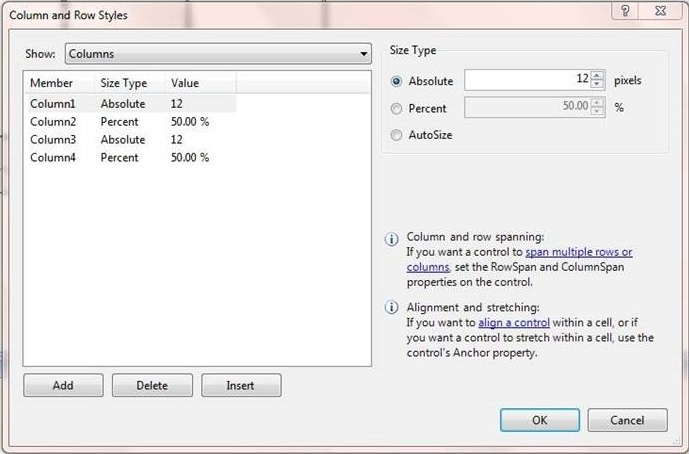The CheckedListbox, Listbox, + 2 Textboxes all have their Dock Property set to DockStyle.Fill, which means they fill their parent container.

Here's the code for the example Project:

```Public Class frmControls

Private Sub frmControls_Load(ByVal sender As System.Object, ByVal e As System.EventArgs) Handles MyBase.Load
'add some items to the checkedListbox + the listbox
clbItems.Items.AddRange(New String() {"One", "Two", "Three", "Four", "Five", "Six", "Seven", "Eight", "Nine", "Ten"})
lbItems.Items.AddRange(New String() {"One", "Two", "Three", "Four", "Five", "Six", "Seven", "Eight", "Nine", "Ten"})
End Sub

Private Sub clbItems_MouseUp(ByVal sender As Object, ByVal e As System.Windows.Forms.MouseEventArgs) Handles clbItems.MouseUp
'depending on the value of chkMultiCheckedItems.Checked
If chkMultiCheckedItems.Checked Then
'display all of the CheckedItems' text in the textbox
txtOutput.Lines = clbItems.CheckedItems.Cast(Of String).ToArray
Else
'display the selectedindex + selecteditem in the textbox
txtOutput.Lines = New String() {String.Format("SelectedIndex: {0}", clbItems.SelectedIndex), _
String.Format("SelectedItem: {0}", If(clbItems.SelectedIndex > -1, clbItems.SelectedItem.ToString, ""))}
End If
End Sub

Private Sub lbItems_SelectedIndexChanged(ByVal sender As System.Object, ByVal e As System.EventArgs) Handles lbItems.SelectedIndexChanged
'depending on the value of chkMultiSelect.Checked
If chkMultiSelect.Checked Then
'display all of the SelectedItems' text in the textbox
txtOutput2.Lines = lbItems.SelectedItems.Cast(Of String).ToArray
Else
'display the selectedindex + selecteditem in the textbox
txtOutput2.Lines = New String() {String.Format("SelectedIndex: {0}", lbItems.SelectedIndex), _
String.Format("SelectedItem: {0}", If(lbItems.SelectedIndex > -1, lbItems.SelectedItem.ToString, ""))}
End If
End Sub

Private Sub chkMultiSelect_CheckedChanged(ByVal sender As System.Object, ByVal e As System.EventArgs) Handles chkMultiSelect.CheckedChanged
If chkMultiSelect.Checked Then
'change the listbox SelectionMode + update the textbox
lbItems.SelectionMode = SelectionMode.MultiSimple
txtOutput2.Lines = lbItems.SelectedItems.Cast(Of String).ToArray
Else
'deselect all SelectedItems + change the listbox SelectionMode
lbItems.SelectedItems.Clear()
lbItems.SelectionMode = SelectionMode.One
End If
End Sub

Private Sub clbItems_SelectedIndexChanged(ByVal sender As Object, ByVal e As System.EventArgs) Handles clbItems.SelectedIndexChanged
If Not chkMultiCheckedItems.Checked Then
'unCheck all checkedItems except the current SelectedIndex
For x As Integer = 0 To clbItems.Items.Count - 1
If x <> clbItems.SelectedIndex Then
clbItems.SetItemChecked(x, False)
End If
Next
End If
End Sub

Private Sub chkMultiCheckedItems_CheckedChanged(ByVal sender As System.Object, ByVal e As System.EventArgs) Handles chkMultiCheckedItems.CheckedChanged
If chkMultiCheckedItems.Checked Then
'display all of the CheckedItems' text in the textbox
txtOutput.Lines = clbItems.CheckedItems.Cast(Of String).ToArray
Else
'unCheck all checkedItems
For x As Integer = 0 To clbItems.Items.Count - 1
clbItems.SetItemChecked(x, False)
Next
'display the selectedindex + selecteditem in the textbox
txtOutput.Lines = New String() {String.Format("SelectedIndex: {0}", clbItems.SelectedIndex), _
String.Format("SelectedItem: {0}", If(clbItems.SelectedIndex > -1, clbItems.SelectedItem.ToString, ""))}
End If
End Sub

End Class
```

## This is the third example Project:I've changed the Form's + all of the Control's Name from the default names.
I've also changed these additional Properties for the Form:

• FormBorderStyle = System.Windows.Forms.FormBorderStyle.FixedSingle
• MaximizeBox = False
• Text = "Reminders"

And this Property for the Listview:

• View = System.Windows.Forms.View.Detail

Then added a column to the Listview.
The Form also has 2 ImageList Components + the Project has 2 images in My.Resources (Project-->Properties-->Resources), that are used dynamically in the code.

Here's the code for the example Project:

```Public Class frmReminder

'reminders index variable
Dim reminders As Integer = 0

<System.Serializable()> _
Public Structure item
Public text As String
Public tag As Object
End Structure

Private Sub frmReminder_FormClosing(ByVal sender As Object, ByVal e As System.Windows.Forms.FormClosingEventArgs) Handles Me.FormClosing
'
'this saves your reminders to a binary file
'using binary serialization
'
Dim items As List(Of item) = (From i As ListViewItem In lvList.Items.Cast(Of ListViewItem)() Select New item With {.text = i.Text, .tag = i.Tag}).ToList
Dim formatter As New Runtime.Serialization.Formatters.Binary.BinaryFormatter
Dim fs As New IO.FileStream("notes.bin", IO.FileMode.Create)
formatter.Serialize(fs, items)
fs.Close()
End Sub

Private Sub frmReminder_Load(ByVal sender As System.Object, ByVal e As System.EventArgs) Handles MyBase.Load
'set some more listview properties
lvList.HideSelection = False
'
'
Dim formatter As New Runtime.Serialization.Formatters.Binary.BinaryFormatter
Dim fs As New IO.FileStream("notes.bin", IO.FileMode.Open)
Dim items As List(Of item) = DirectCast(formatter.Deserialize(fs), Global.System.Collections.Generic.List(Of item))
fs.Close()
For Each i In items
lvList.Items(lvList.Items.Count - 1).Text = i.text
lvList.Items(lvList.Items.Count - 1).Tag = i.tag
Next
lvList.SelectedIndices.Clear()
End Sub

Private Sub btnAdd_Click(ByVal sender As System.Object, ByVal e As System.EventArgs) Handles btnAdd.Click
'create the listview index images. standard + wide sizes
Dim img As New Bitmap(My.Resources.blank)
Dim imgWide As New Bitmap(My.Resources.blankWide)
Dim gr() As Graphics = {Graphics.FromImage(img), Graphics.FromImage(imgWide)}
Dim textSize As SizeF = gr(0).MeasureString(reminders.ToString, Me.Font)
gr(0).DrawString(reminders.ToString, Me.Font, Brushes.Black, (img.Width - textSize.Width) / 2, (img.Height - textSize.Height) / 2)
gr(1).DrawString(reminders.ToString, Me.Font, Brushes.Black, (imgWide.Width - textSize.Width) / 2, (imgWide.Height - textSize.Height) / 2)
'add the standard image to imageList1
'add the wide image to imageList2
'set the listview SmallImageList property
If reminders >= 10 Then
lvList.SmallImageList = ImageList2
Else
lvList.SmallImageList = ImageList1
End If
'increment the reminders variable
reminders += 1
'add the reminder to the listview
lvList.Invalidate()
lvList.Items.Add("{New Reminder" & reminders.ToString & "}", ImageList2.Images.Count - 1)
'select the new reminder
lvList.Items(lvList.Items.Count - 1).Selected = True
End Sub

Private Sub btnRemove_Click(ByVal sender As System.Object, ByVal e As System.EventArgs) Handles btnRemove.Click
'remove item
If lvList.SelectedItems.Count = 1 Then
'get the index of the SelectedItem
Dim index As Integer = lvList.SelectedIndices(0)
'remove the SelectedItem
lvList.Items.RemoveAt(index)
'rearrange the listview images
For x As Integer = 0 To lvList.Items.Count - 1
lvList.Items(x).ImageIndex = x
Next
'remove the last image from both imageLists
ImageList2.Images.RemoveAt(ImageList2.Images.Count - 1)
ImageList1.Images.RemoveAt(ImageList1.Images.Count - 1)
'decrement the reminders variable
reminders -= 1
'set the listview SmallImageList property
If reminders > 10 Then
lvList.SmallImageList = ImageList2
Else
lvList.SmallImageList = ImageList1
End If
'depending on the index of the removed item
'select either the preceding item or the next item
lvList.Invalidate()
If lvList.Items.Count > 0 Then
If index < lvList.Items.Count Then
lvList.Items(index).Selected = True
Else
lvList.Items(index - 1).Selected = True
End If
End If
End If
End Sub

Private Sub btnMoveUp_Click(ByVal sender As System.Object, ByVal e As System.EventArgs) Handles btnMoveUp.Click
'move up
'remove the selectedItem from the list + reinsert 1 place higher
Dim index As Integer = lvList.SelectedIndices(0)
Dim text As String = lvList.Items(index).Text
Dim tag As Object = lvList.Items(index).Tag
lvList.Items.RemoveAt(index)
lvList.Items.Insert(index - 1, text)
lvList.Items(index - 1).Tag = tag
'rearrange the listview images
For x As Integer = 0 To lvList.Items.Count - 1
lvList.Items(x).ImageIndex = x
Next
'select the newly inserted reminder
lvList.Items(index - 1).Selected = True
End Sub

Private Sub btnMoveDown_Click(ByVal sender As System.Object, ByVal e As System.EventArgs) Handles btnMoveDown.Click
'move down
'remove the selectedItem from the list + reinsert 1 place lower
Dim index As Integer = lvList.SelectedIndices(0)
Dim text As String = lvList.Items(index).Text
Dim tag As Object = lvList.Items(index).Tag
lvList.Items.RemoveAt(index)
lvList.Items.Insert(index + 1, text)
lvList.Items(index + 1).Tag = tag
'rearrange the listview images
For x As Integer = 0 To lvList.Items.Count - 1
lvList.Items(x).ImageIndex = x
Next
'select the newly inserted reminder
lvList.Items(index + 1).Selected = True
End Sub

Private Sub lvList_SelectedIndexChanged(ByVal sender As Object, ByVal e As System.EventArgs) Handles lvList.SelectedIndexChanged
If lvList.SelectedItems.Count = 1 Then
'enable/disable the buttons depending on the SelectedItems.Count
'+ the position of the SelectedItem in the list
btnMoveUp.Enabled = lvList.SelectedIndices(0) > 0
btnMoveDown.Enabled = lvList.SelectedIndices(0) < lvList.Items.Count - 1
btnRemove.Enabled = True
txtNote.Enabled = True
'get the tag property from the SelectedItem + display in the textbox
Dim txtObject As Object = lvList.Items(lvList.SelectedIndices(0)).Tag
txtNote.Text = If(txtObject IsNot Nothing, txtObject.ToString, "")
Else
'disable all of the buttons (except btnAdd) + clear the textbox + disable it
btnMoveUp.Enabled = False
btnMoveDown.Enabled = False
btnRemove.Enabled = False
txtNote.Enabled = False
txtNote.Text = ""
End If
End Sub

Private Sub txtNote_TextChanged(ByVal sender As System.Object, ByVal e As System.EventArgs) Handles txtNote.TextChanged
If lvList.SelectedItems.Count = 1 Then
'save txtNote.Text to the lvList.SelectedItem's tag property
Dim index As Integer = lvList.SelectedIndices(0)
lvList.Items(index).Tag = txtNote.Text
End If
End Sub

End Class```

## This is the fourth example Project (Using a Splashscreen + Extension methods):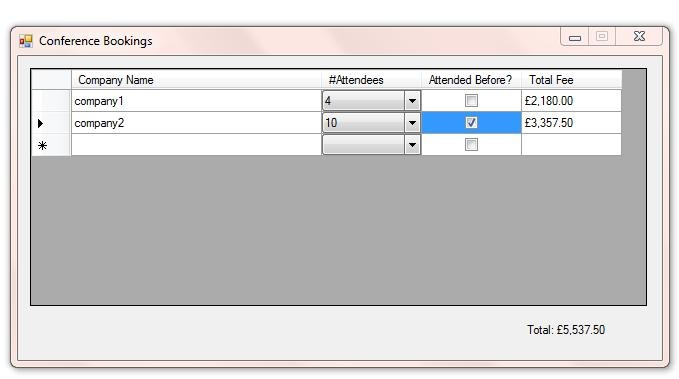I've changed the Form's + all of the Control's Name from the default names.
I've also changed these additional Properties for the Form:

• FormBorderStyle = System.Windows.Forms.FormBorderStyle.FixedSingle
• MaximizeBox = False
• Text = "Conference Bookings"

Also, I added 4 columns (a TextboxColumn, a ComboboxColumn, a CheckboxColumn, + another TextboxColumn) to the Datagridview. The last (TextboxColumn) is Readonly.

This is the code for the main Form:

```Public Class frmInput

'this boolean flag variable is used to avoid calculating the total before the form is loaded

'this is a form level array, so you only declare + load it once
'arrays declared locally within a procedure are redeclared every time the procedure runs
Dim values() As Integer = {0, 695, 545, 545, 545, 480, 480, 480, 480, 395, 395, 395, 395, 395, 395, 395, 395}

Private Sub frmInput_FormClosing(ByVal sender As Object, ByVal e As System.Windows.Forms.FormClosingEventArgs) Handles Me.FormClosing
'save datagridview contents
dgvInput.saveToXML("data.xml") 'user defined extension method
End Sub

Private Sub frmInput_Load(ByVal sender As System.Object, ByVal e As System.EventArgs) Handles MyBase.Load
'bind the datagridviewcombobox column
Dim dgvComboboxes As DataGridViewComboBoxColumn = DirectCast(dgvInput.Columns(1), DataGridViewComboBoxColumn)
dgvComboboxes.DataSource = New String() {"1", "2", "3", "4", "5", "6", "7", "8", "9", "10", "11", "12", "13", "14", "15", "16"}
End Sub

Private Sub DGVComboIndexChanged(ByVal sender As System.Object, ByVal e As System.EventArgs)
'this handles the datagridviewcombobox cell selectedindexchanged event
Dim cb As ComboBox = DirectCast(sender, ComboBox)
'calculate the cost of 1 company sending x amount of employees to conference
calculateCost(dgvInput.CurrentCell.RowIndex, cb)
'calculate the total for all rows in the datagridview
calculateTotal()
End Sub

Private Sub dgvInput_CellValueChanged(ByVal sender As Object, ByVal e As System.Windows.Forms.DataGridViewCellEventArgs) Handles dgvInput.CellValueChanged
'if sender is the checkbox column
If e.ColumnIndex = 2 Then
'calculate the cost of 1 company sending x amount of employees to conference
calculateCost(e.RowIndex)
'calculate the total for all rows in the datagridview
calculateTotal()
End If
End If
End Sub

Private Sub dgvInput_CurrentCellDirtyStateChanged(ByVal sender As Object, ByVal e As System.EventArgs) Handles dgvInput.CurrentCellDirtyStateChanged
'if CurrentCell is the checkbox column
'this is necessary to trigger the dgvInput_CellValueChanged event
If dgvInput.CurrentCell.ColumnIndex = 2 Then
dgvInput.CommitEdit(DataGridViewDataErrorContexts.Commit)
End If
End Sub

Private Sub dgvInput_EditingControlShowing(ByVal sender As Object, _
ByVal e As System.Windows.Forms.DataGridViewEditingControlShowingEventArgs) Handles dgvInput.EditingControlShowing
' Attempt to cast the EditingControl to a ComboBox.
'this will only work if CurrentCell is the combobox column
Dim cb As ComboBox = TryCast(e.Control, ComboBox)
'if it is the combobox column...
If cb IsNot Nothing Then
End If
End Sub

'calculate the total for all rows in the datagridview
calculateTotal()
End Sub

Private Sub dgvInput_RowsRemoved(ByVal sender As Object, ByVal e As System.Windows.Forms.DataGridViewRowsRemovedEventArgs) Handles dgvInput.RowsRemoved
'calculate the total for all rows in the datagridview
calculateTotal()
End Sub

Private Sub calculateCost(ByVal rowIndex As Integer, Optional ByVal cb As ComboBox = Nothing)
If cb Is Nothing Then
'if the optional cb parameter is omitted...
'the formula is number of attendees * cost for that many attendees,
'which is stored in the form level values() array
dgvInput(3, rowIndex).Value = If(dgvInput(2, rowIndex).Value IsNot Nothing AndAlso CBool(dgvInput(2, rowIndex).Value) = True, _
((CInt(dgvInput(1, rowIndex).Value) * values(CInt(dgvInput(1, rowIndex).Value))) * 0.85).ToString("c2"), _
(CInt(dgvInput(1, rowIndex).Value) * values(CInt(dgvInput(1, rowIndex).Value))).ToString("c2"))
Else
dgvInput(3, rowIndex).Value = If(dgvInput(2, rowIndex).Value IsNot Nothing AndAlso CBool(dgvInput(2, rowIndex).Value) = True, _
((CInt(cb.SelectedItem) * values(CInt(cb.SelectedItem))) * 0.85).ToString("c2"), _
(CInt(cb.SelectedItem) * values(CInt(cb.SelectedItem))).ToString("c2"))
End If
End Sub

Private Sub calculateTotal()
'this totals the datagridview rows (Total Fee column)
Dim total = (From row As DataGridViewRow In dgvInput.Rows.Cast(Of DataGridViewRow)() _
Select If(row.IsNewRow OrElse row.Cells(3).Value Is Nothing, 0, CDec(row.Cells(3).Value.ToString))).Sum
'+ displays the total in lblTotal formatted as a decimal
'in your local currency with 2 decimal places
lblTotal.Text = String.Format("Total: {0:c2}", total)
End Sub

End Class
```

The timing for the Splashscreen duration + fading is controlled by 2 Timer Components.
To create a Splashscreen from a standard Form, there are Properties you'll need to set:

• ControlBox = False
• FormBorderStyle = System.Windows.Forms.FormBorderStyle.None
• MaximizeBox = False
• MinimizeBox = False
• StartPosition = System.Windows.Forms.FormStartPosition.CenterScreen

But if you use a template, most of the Properties are setup already.

Here's the code for the Splashscreen:

```Public NotInheritable Class frmSplashScreen

Private Sub frmSplashScreen_Load(ByVal sender As Object, ByVal e As System.EventArgs) Handles Me.Load
'this makes all parts of the form that are red, transparent
'but it's still got red parts as VB doesn't display colors perfectly some times -
'as there are 256^3 colors in vb and the color has to be exactly the same as the TransparencyKey.
'you can use any form as a splashscreen, either the standard splashscreen
Me.TransparencyKey = Color.Red
End Sub

Private Sub Timer1_Tick(ByVal sender As System.Object, ByVal e As System.EventArgs) Handles Timer1.Tick
'after 3 seconds, timer1 ticks, then disables itself + starts timer2
Timer1.Enabled = False
Timer2.Enabled = True
End Sub

Private Sub Timer2_Tick(ByVal sender As System.Object, ByVal e As System.EventArgs) Handles Timer2.Tick
Me.Opacity -= 0.1
If Me.Opacity = 0 Then
frmInput.Show()
Me.Close()
End If
End Sub

End Class```

This Splashscreen is partially transparent at runtime.

This Project uses Extension methods. 2 methods are added to the Datagridview control. These apply to any instance of a Datagridview in the Project.

Here's the code for the Extension methods:

```''' <summary>
''' extensions module
''' </summary>
''' <remarks>these are extensions for the datagridview.
''' ideally you should only create reusable extension methods
''' whereas these are specialized, but they demonstrate the technique</remarks>
Module extensions

<System.Runtime.CompilerServices.Extension()> _
Public Sub saveToXML(ByVal instance As DataGridView, ByVal xmlFilename As String)
'in an extension method the first parameter refers to the class being extended.
'this creates + saves an xml file using the datagridview rows + the xmlFilename specified
Dim xml = _
<?xml version="1.0" standalone="no"?>
<DGV>
</DGV>
For x As Integer = 0 To instance.Rows.Count - 1
If Not instance.Rows(x).IsNewRow Then
If instance.Rows(x).Cells(0).Value Is Nothing Then Continue For
<DataGridViewRow>
<Value1><%= instance.Rows(x).Cells(0).Value.ToString %></Value1>
<Value2><%= If(instance.Rows(x).Cells(1).Value Is Nothing, "", instance.Rows(x).Cells(1).Value.ToString) %></Value2>
<Value3><%= If(instance.Rows(x).Cells(2).Value Is Nothing, CStr(False), instance.Rows(x).Cells(2).Value.ToString) %></Value3>
<Value4><%= If(instance.Rows(x).Cells(3).Value Is Nothing, "", instance.Rows(x).Cells(3).Value.ToString) %></Value4>
</DataGridViewRow>)
End If
Next
xml.Save(xmlFilename)
End Sub

<System.Runtime.CompilerServices.Extension()> _
Public Sub loadFromXML(ByVal instance As DataGridView, ByVal xmlFilename As String)
If Not IO.File.Exists(xmlFilename) Then Return
'this extension method reads the specified xml file + loads the values into the DataGridView
Dim xml As XDocument = XDocument.Load(xmlFilename)
Dim rows = (From node In xml...<DataGridViewRow> _
Select New Object() {node...<Value1>.Value, _
node...<Value2>.Value, _
node...<Value3>.Value, _
node...<Value4>.Value}).ToArray
For Each r In rows
Next
End Sub

End Module```

## This is the fifth example Project (Extending Controls):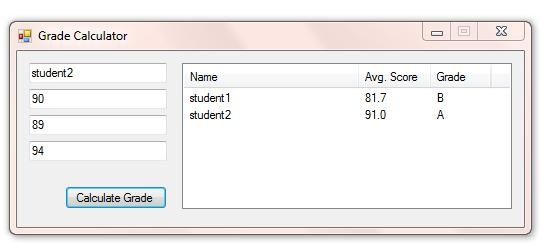I've changed the Form's + all of the Control's Name from the default names.
I've also changed these additional Properties for the Form:

• FormBorderStyle = System.Windows.Forms.FormBorderStyle.FixedSingle
• MaximizeBox = False

And this Property for the Listview:

• View = System.Windows.Forms.View.Detail

Then added 3 columns to the Listview + changed their ColumnHeader Text. The promptTextboxes have a prompt Property + an isInteger Property that you need to set too.

Here's the code for the example Project:

```Public Class frmGrades
'form level array
'only needs to be declared + loaded once in lifetime of form

'initialize the promptTextboxes
ptbName.init()
ptbExamScore1.init()
ptbExamScore2.init()
ptbCourseWorkScore.init()
grades = New Object(,) {{100, "A+"}, {95, "A"}, {85, "B"}, {75, "C"}, {65, "D"}, {55, "E"}, {50, "U"}}
End Sub

Private Sub btnCalculateGrade_Click(ByVal sender As System.Object, ByVal e As System.EventArgs) Handles btnCalculateGrade.Click
'declare + load new array of promptTextboxes
Dim ptbs() As promptTextbox = {ptbExamScore1, ptbExamScore2, ptbCourseWorkScore}
'calculate average score from promptTextbox values
Dim average As Double = ptbs.Average(Function(p As promptTextbox) p.score)
firstList.RemoveAll(Function(s) s = "")
Dim grade As String = firstList.LastOrDefault
End Sub

End Class```

This is the code for the Extended Textbox Control:

```''' <summary>
''' extended textbox control
''' </summary>
''' <remarks></remarks>
Public Class promptTextbox
Inherits TextBox

'class level variable - available throughout the class
Dim oldText As String

'class level constant
Const WM_PASTE As Integer = 770

Protected Overrides Sub OnKeyDown(ByVal e As System.Windows.Forms.KeyEventArgs)
'don't allow arrow keys
If e.KeyCode = Keys.Left OrElse e.KeyCode = Keys.Up OrElse e.KeyCode = Keys.Right OrElse e.KeyCode = Keys.Down Then
e.SuppressKeyPress = True
End If
MyBase.OnKeyDown(e)
'set variable used in OnKeyUp procedure
oldText = MyBase.Text
End Sub

Protected Overrides Sub OnKeyUp(ByVal e As System.Windows.Forms.KeyEventArgs)
'handles Delete + Back Keys
'to determine whether to display prompt or not
If e.KeyCode = Keys.Delete Then
showHidePrompt(oldText, MyBase.Text.Remove(MyBase.SelectionStart, MyBase.SelectionLength))
ElseIf e.KeyCode = Keys.Back Then
Dim newText As String = ""
If MyBase.SelectionLength > 0 Then
newText = MyBase.Text.Remove(MyBase.SelectionStart, MyBase.SelectionLength)
Else
If MyBase.SelectionStart > 0 Then
newText = MyBase.Text.Remove(MyBase.SelectionStart - 1, 1)
End If
End If
showHidePrompt(oldText, newText)
End If
MyBase.OnKeyUp(e)
End Sub

Protected Overrides Sub OnKeyPress(ByVal e As System.Windows.Forms.KeyPressEventArgs)
If Not Char.IsControl(e.KeyChar) Then
'check if prompt should be hidden
Dim newText As String
If MyBase.SelectedText <> "" Then
newText = MyBase.Text.Replace(prompt, "").Replace(MyBase.SelectedText, "").Insert(MyBase.SelectionStart, e.KeyChar)
Else
newText = MyBase.Text.Replace(prompt, "").Insert(MyBase.SelectionStart, e.KeyChar)
End If
showHidePrompt(MyBase.Text, newText)
'if control is being used as an integer textbox...
If isInteger Then
'disallow non integer input
e.Handled = If(newText = "", True, Not Integer.TryParse(newText, _score))
'set score property
_score = If(MyBase.Text = "", 0, score)
End If
End If
MyBase.OnKeyPress(e)
End Sub

Protected Overrides Sub OnMouseDown(ByVal e As System.Windows.Forms.MouseEventArgs)
'select all text if text = prompt
If MyBase.Text = prompt Then MyBase.SelectAll()
MyBase.OnMouseDown(e)
End Sub

Protected Overrides Sub OnLeave(ByVal e As System.EventArgs)
'check if prompt should be shown
showHidePrompt(MyBase.Text, MyBase.Text)
MyBase.OnLeave(e)
End Sub

Protected Overrides Sub WndProc(ByRef m As System.Windows.Forms.Message)
'don't allow pasting
If m.Msg = WM_PASTE Then Return
MyBase.WndProc(m)
End Sub

Private Sub showHidePrompt(ByVal previousText As String, ByVal newText As String)
'show or hide the prompt depending on the previous text in the control + the new text in the control
'when the control shows prompt it changes forecolor + selects all text
'when the prompt is hidden it changes forecolor to Color.Black
If previousText = prompt And Not newText = prompt Then
MyBase.ForeColor = Color.Black
Else
If newText = "" Then
MyBase.Text = prompt
MyBase.ForeColor = SystemColors.ControlDark
MyBase.SelectAll()
End If
End If
End Sub

Public Sub init()
'shows prompt
showHidePrompt(MyBase.Text, MyBase.Text)
End Sub

''' <summary>
''' prompt property
''' </summary>
''' <remarks>what is displayed as a prompt</remarks>
Private _prompt As String
Public Property prompt() As String
Get
Return _prompt
End Get
Set(ByVal value As String)
_prompt = value
End Set
End Property

''' <summary>
''' score property
''' </summary>
''' <remarks>used when control is used as an integer textbox</remarks>
Private _score As Integer
Public ReadOnly Property score() As Integer
Get
Return _score
End Get
End Property

''' <summary>
''' isInteger property
''' </summary>
''' <remarks>determines whether to allow only integers or any text</remarks>
Private _isInteger As Boolean
Public Property isInteger() As Boolean
Get
Return _isInteger
End Get
Set(ByVal value As Boolean)
_isInteger = value
End Set
End Property

End Class```

## This is the sixth example Project (Creating UserControls):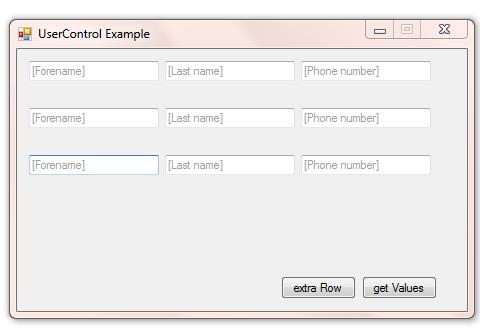I've changed the Form's + all of the Control's Name from the default names.
I've also changed these additional Properties for the Form:

• FormBorderStyle = System.Windows.Forms.FormBorderStyle.FixedSingle
• MaximizeBox = False
• Text = "UserControl Example"

The UserControl has 3 promptTextboxes (although you can use any Controls). For the promptTextboxes (as demonstrated in the last example) you need to set this Property:

• prompt = "[Forename]" (for example)

This is the main Form code:

```Public Class frmDemo

Private Sub btnNewRow_Click(ByVal sender As System.Object, ByVal e As System.EventArgs) Handles btnNewRow.Click
'this adds new dynamic ctrlRow control to the panel
Dim cr As New ctrlRow
cr.Left = 0
cr.Top = (Me.pnlMain.Controls.OfType(Of ctrlRow).Count * cr.Height) + pnlMain.AutoScrollPosition.Y
End Sub

Private Sub btnGetValues_Click(ByVal sender As System.Object, ByVal e As System.EventArgs) Handles btnGetValues.Click
'this loops through all of the ctrlRow controls on the panel + returns
'their foreName, lastName, + phoneNumber properties
For Each cr As ctrlRow In Me.pnlMain.Controls.OfType(Of ctrlRow)()
MsgBox(String.Format("ForeName: {0} LastName: {1}   Phone Number: {2}", cr.foreName, cr.lastName, cr.phoneNumber))
Next
End Sub

End Class```

This is the UserControl code:

```Public Class ctrlRow

''' <summary>
''' foreName property
''' </summary>
''' <remarks>this exposes ptbForeName.Text</remarks>
Private _foreName As String
Public ReadOnly Property foreName() As String
Get
Return _foreName
End Get
End Property

''' <summary>
''' lastName property
''' </summary>
''' <remarks>this exposes ptbLastName.Text</remarks>
Private _lastName As String
Public ReadOnly Property lastName() As String
Get
Return _lastName
End Get
End Property

''' <summary>
''' phoneNumber property
''' </summary>
''' <remarks>this exposes ptbPhone.Text</remarks>
Private _phoneNumber As String
Public ReadOnly Property phoneNumber() As String
Get
Return _phoneNumber
End Get
End Property

Private Sub ptbForeName_TextChanged(ByVal sender As System.Object, ByVal e As System.EventArgs) Handles ptbForeName.TextChanged
'set foreName property
_foreName = If(ptbForeName.Text <> ptbForeName.prompt, ptbForeName.Text, "")
End Sub

Private Sub ptbLastName_TextChanged(ByVal sender As System.Object, ByVal e As System.EventArgs) Handles ptbLastName.TextChanged
'set lastName property
_lastName = If(ptbLastName.Text <> ptbLastName.prompt, ptbLastName.Text, "")
End Sub

Private Sub ptbPhone_TextChanged(ByVal sender As System.Object, ByVal e As System.EventArgs) Handles ptbPhone.TextChanged
'set phoneNumber property
_phoneNumber = If(ptbPhone.Text <> ptbPhone.prompt, ptbPhone.Text, "")
End Sub

Public Sub New()
' This call is required by the Windows Form Designer.
InitializeComponent()
'initialize the promptTextboxes
ptbForeName.init()
ptbLastName.init()
ptbPhone.init()
End Sub

End Class```

## This is the seventh example Project (Responding to Form Events):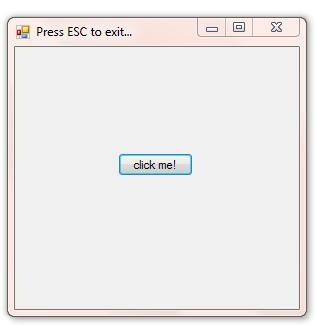This is a simple example that shows how to intercept + respond to Form Events. Moving the MousePointer towards the Button causes the Button to be moved.
This example uses a Form + 3 Classes, which I haven't mentioned before. Classes are a way to structure your code into manageable reusable Components.

This is the Form code:

```Public Class frmDemo

Const minimumDistance As Integer = 50 '50 pixels

Private Sub Form1_KeyDown(ByVal sender As Object, ByVal e As System.Windows.Forms.KeyEventArgs) Handles Me.KeyDown
'if ESC then close app.
If e.KeyCode = Keys.Escape Then Me.Close()
End Sub

Private Sub Form1_Load(ByVal sender As Object, ByVal e As System.EventArgs) Handles Me.Load
'this gives the form a first look at the keyboard input
Me.KeyPreview = True
End Sub

Private Sub Form1_MouseMove(ByVal sender As Object, ByVal e As System.Windows.Forms.MouseEventArgs) Handles Me.MouseMove
'if the pousePosition is less than 50 pixels from the centre
'of the button in any direction, the button is relocated
Dim buttonCentre As Point = btnCantClick.Location
buttonCentre.Offset(btnCantClick.Width \ 2, btnCantClick.Height \ 2)
If measurement.lineLength(buttonCentre, e.Location) < minimumDistance Then
Dim angleRadians As Single = CSng(Math.PI * (angles.FindAngle(buttonCentre, e.Location)) / 180)
'Calculate X2 and Y2
Dim pointX2 As Integer = CInt(e.Location.X - Math.Sin(angleRadians) * minimumDistance)
Dim pointY2 As Integer = CInt(e.Location.Y + Math.Cos(angleRadians) * minimumDistance)
Dim newLocation As New Point(pointX2, pointY2)
newLocation.Offset(-(btnCantClick.Width \ 2), -(btnCantClick.Height \ 2))
btnCantClick.Location = newLocation
End If
End Sub

Private Sub btnCantClick_Click(ByVal sender As System.Object, ByVal e As System.EventArgs) Handles btnCantClick.Click
MessageBox.Show("Congratulations! You found the loophole...")
End Sub

End Class```

These are the Classes:

The angles Class:

```Public Class angles

''' <summary>
''' returns degrees in this coordinate system:
''' N,E,S,W = 0,90,180,270 degrees
''' </summary>
''' <param name="p1">point1</param>
''' <param name="p2">point2</param>
''' <returns></returns>
''' <remarks></remarks>
Public Shared Function FindAngle(ByVal p1 As Point, ByVal p2 As Point) As Integer

Dim atn1 As Double = Math.Atan(1)
Dim dx As Double = p2.X - p1.X
Dim dy As Double = p2.Y - p1.Y

'Avoid divide by 0 error
If (dx = 0) Then dx = 1.0E-20

'Find arctangent and (orient to 12 o'clock)
Dim angle As Double = Math.Atan(dy / dx) + (atn1 * 2)

If (p2.X < p1.X) Then angle = angle + (atn1 * 4)

Return CInt(angle * 45 / atn1)

End Function

End Class
```

The measurement Class:

```Public Class measurement

''' <summary>
''' lineLength
''' </summary>
''' <param name="p1">point1</param>
''' <param name="p2">point2</param>
''' <returns>length in pixels between p1 and p2</returns>
''' <remarks></remarks>
Public Shared Function lineLength(ByVal p1 As Point, ByVal p2 As Point) As Integer
Return CInt(Math.Sqrt(Math.Abs(p1.X - p2.X) ^ 2 + Math.Abs(p1.Y - p2.Y) ^ 2))
End Function

End Class```

The rounding Class:

```Public Class rounding

''' <summary>
''' toInteger
''' </summary>
''' <param name="number"></param>
''' <returns>an integer</returns>
''' <remarks>rounds up or down using midpoint rounding</remarks>
Public Shared Function toInteger(ByVal number As Double) As Integer
Return CInt(Math.Round(number, 0, MidpointRounding.ToEven))
End Function

End Class```